NEET  >  Test: Colligative Properties (Lowering Of Vapour Pressure)

# Test: Colligative Properties (Lowering Of Vapour Pressure)

Test Description

## 20 Questions MCQ Test Chemistry Class 12 | Test: Colligative Properties (Lowering Of Vapour Pressure)

Test: Colligative Properties (Lowering Of Vapour Pressure) for NEET 2023 is part of Chemistry Class 12 preparation. The Test: Colligative Properties (Lowering Of Vapour Pressure) questions and answers have been prepared according to the NEET exam syllabus.The Test: Colligative Properties (Lowering Of Vapour Pressure) MCQs are made for NEET 2023 Exam. Find important definitions, questions, notes, meanings, examples, exercises, MCQs and online tests for Test: Colligative Properties (Lowering Of Vapour Pressure) below.
Solutions of Test: Colligative Properties (Lowering Of Vapour Pressure) questions in English are available as part of our Chemistry Class 12 for NEET & Test: Colligative Properties (Lowering Of Vapour Pressure) solutions in Hindi for Chemistry Class 12 course. Download more important topics, notes, lectures and mock test series for NEET Exam by signing up for free. Attempt Test: Colligative Properties (Lowering Of Vapour Pressure) | 20 questions in 30 minutes | Mock test for NEET preparation | Free important questions MCQ to study Chemistry Class 12 for NEET Exam | Download free PDF with solutions
 1 Crore+ students have signed up on EduRev. Have you?
Test: Colligative Properties (Lowering Of Vapour Pressure) - Question 1

### This section contains 12 multiple choice questions. Each question has four choices (a), (b), (c) and (d), out of which ONLY ONE is correct The vapour pressure of pure liquid solvent is 0.80 atm. When a non-volatile solute B is added to the solvent, its vapour pressure drops to 0.60 atm. Thus, mole fraction of the component B is

Detailed Solution for Test: Colligative Properties (Lowering Of Vapour Pressure) - Question 1

Using Raoults law for lowering of vapour pressure.We get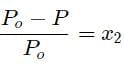putting,Values of actual and lowered vapour pressure we get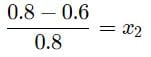where x2​ is the mole fraction .

Test: Colligative Properties (Lowering Of Vapour Pressure) - Question 2

### Vapour pressure of pure water at 298 K is 23.8 torr. Thus, vapour pressure of a solution containing 6 g urea and 68.4 g sucrose in 10 moles of water is

Detailed Solution for Test: Colligative Properties (Lowering Of Vapour Pressure) - Question 2

By Raoult’s law, for a mixture of non-volatile solute in volatile solvent.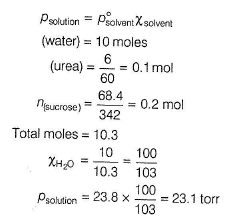Test: Colligative Properties (Lowering Of Vapour Pressure) - Question 3

### Vapour pressure of nitrobenzene (molar mass = 123 g mol-1) is 3.6 kNm-2 and that of water (molar mass = 18 g mol-1) is 977 kNm-2. They form immiscible mixture at the given temperature. Thus, percentage of nitrobenzene in the vapour phase is

Detailed Solution for Test: Colligative Properties (Lowering Of Vapour Pressure) - Question 3

For immiscible solvents, composition of each component is based on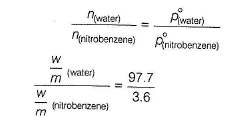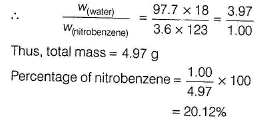Test: Colligative Properties (Lowering Of Vapour Pressure) - Question 4

The vapour pressure of n-hexane at 350 K is 840 torr and that of cyclohexane is 600 torr. Mole fraction of hexane in the mixture that boils at 350 K and 1 atm pressure assuming ideal behaviour is

Detailed Solution for Test: Colligative Properties (Lowering Of Vapour Pressure) - Question 4

d) Mixture boils at 1 atm and 350 K.
Thus, total vapour pressure is 1 atm = 760torr (h) used for hexane and (ch) for cyclohexane.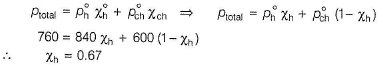Test: Colligative Properties (Lowering Of Vapour Pressure) - Question 5

At 298 K, the vapour pressure of pure water is 23.76 mm Hg and that of an aqueous solution is 22.98 mm Hg. Thus, molality of solution is (assume dilute solution)

Detailed Solution for Test: Colligative Properties (Lowering Of Vapour Pressure) - Question 5

(c)

By Raoult’s law for a non-volatile solute in volatile solvent.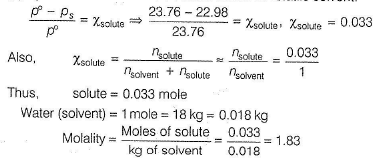Test: Colligative Properties (Lowering Of Vapour Pressure) - Question 6

A binary liquid solution is prepared by mixing n-heptane and ethanol. Which one of the following statements is/are correct regarding the behaviour of the solution?

Detailed Solution for Test: Colligative Properties (Lowering Of Vapour Pressure) - Question 6

Ethanol molecules are joined to each other by intermolecular H-bonding thus, they have high boiling point. When n-hexane is mixed into it, H-bonding breaks. Since, force of attraction between n-hexane and ethanol molecules is smaller than between the hexane-hexane and ethanol-ethanol. Thus, mixture is more volatile. This shows positive deviation from Raoult’s law

Test: Colligative Properties (Lowering Of Vapour Pressure) - Question 7

18 g of glucose (C6H12O6 ) is added to 178.2 g of water. The vapour pressure of water for this aqueous solution at 100°C is

Detailed Solution for Test: Colligative Properties (Lowering Of Vapour Pressure) - Question 7

(d)

At 100°C, vapour pressure of water = 1 atm. Thus, p0 = 760torr Let vapour pressure of the solution = ps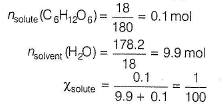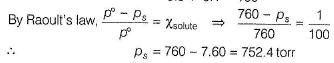Test: Colligative Properties (Lowering Of Vapour Pressure) - Question 8

Which of the following liquid pairs shows a positive deviation from Raoult’s law?

Detailed Solution for Test: Colligative Properties (Lowering Of Vapour Pressure) - Question 8

(a) When HCI is mixed with H20, force of attraction between HCI and H2O  molecules is increased (due to dissolution), hence vaporisation decreased, a case of negative deviation.

(b) CH3OH molecules are joined by intermolecular H-bonding which provides it a liquid state. On adding benzene, H-bonding breaks and force of attraction between CH3OH and benzene molecules is less than between CH3OH-CH3OH and between benzene-benzene hence, more vaporisation of mixture, a case of positive deviation.

(c) As in (a), negative deviation.

(d) CH3COCH3 and CHCI3 are volatile. On mixing, there is increase in intermolecular H-bonding due to the formation of chloretone (A)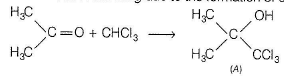Thus, force of attraction between (CHCI3-CH3OCH3) molecules is larger than between (CHCI3-CHCI3)and (CH3COCH3 - CH3OC H3) molecules. Thus, vaporisation decreases, a case of negative deviation.

Test: Colligative Properties (Lowering Of Vapour Pressure) - Question 9

A solution of two liquids boils at a temperature more than the boiling point of either of them. Hence, the binary solution shows

Detailed Solution for Test: Colligative Properties (Lowering Of Vapour Pressure) - Question 9

If force of attraction between A and B (solvents) is higher than that between A and A or B and B, then there is decrease in vaporisation.
Thus, higher temperature (i.e. boiling point) is required to convert liquids into vapour state. This is a case of negative deviation from Raoult's law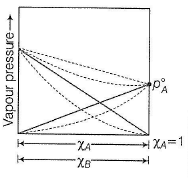Test: Colligative Properties (Lowering Of Vapour Pressure) - Question 10

Lowering of vapour pressure of an aqueous solution of a non-volatile non-electrolyte 1 molal aqueous solution at 100°C is

Detailed Solution for Test: Colligative Properties (Lowering Of Vapour Pressure) - Question 10

(c) Molality = 1 mol kg-1 (solvent) Thus, solute = 1 mole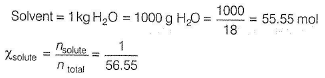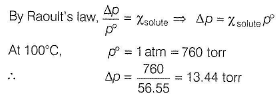Test: Colligative Properties (Lowering Of Vapour Pressure) - Question 11

Which combination of following terms is matched correctly?
I. Vapour pressure

II. Intermolecular forces

III. Latent heat of vaporisation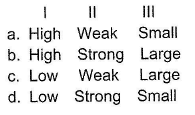Detailed Solution for Test: Colligative Properties (Lowering Of Vapour Pressure) - Question 11

(a) Liquid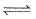Vapour

(a) If vapour pressure is high, it means equilibrium is in forward side. This is attained when intermolecular forces holding the molecules are of weak nature. Low heat is required to convert liquid into vapour. Thus, (a) is correct.

(b) Thus, incorrect.

(c) When vapour pressure is low, it means intermolecular forces are strong hence (c) is incorrect.

(d) When vapour pressure is low, intermolecular forces are strong, hence large heat is required thus, (d) is incorrect,

Test: Colligative Properties (Lowering Of Vapour Pressure) - Question 12

The solubility of urea in methanol is 17 g urea/100 mL methanol. Density of methanol is 0.792 g/mL at 293 K at which vapour pressure of methanol is 96.0 mm of Hg. Thus, vapour pressure of saturated solution of urea in methanol is

Detailed Solution for Test: Colligative Properties (Lowering Of Vapour Pressure) - Question 12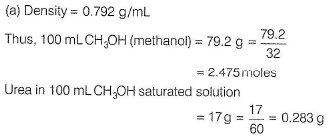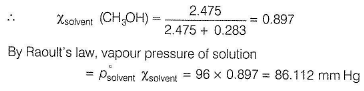*Multiple options can be correct
Test: Colligative Properties (Lowering Of Vapour Pressure) - Question 13

One or More than One Options Correct Type
This section contains 3 multiple type questions. Each question has 4 choices (a), (b), (c) and (d), out of which ONE or MORE THAN ONE are correct.

Q. Benzene and naphthalene form an ideal solution at room temperature. For this process, the true statement(s) is/are  [JEE Advanced 2013]

Detailed Solution for Test: Colligative Properties (Lowering Of Vapour Pressure) - Question 13

When binary mixture is ideal, then by Raoult’s law

ΔV = 0, ΔHmix = 0, no enthalpy change

Mixing is spontaneous, thus ΔG < 0 By thermodynamic property,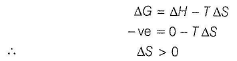Thus, on mixing, entropy increases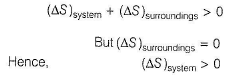*Multiple options can be correct
Test: Colligative Properties (Lowering Of Vapour Pressure) - Question 14

Total vapour pressure of mixture of 1 mole of a volatile component A (p°A = 100 mm Hg) and 3 moles of volatile component B(p°B = 60 mm Hg) is 75 mm Hg. For such case,

Detailed Solution for Test: Colligative Properties (Lowering Of Vapour Pressure) - Question 14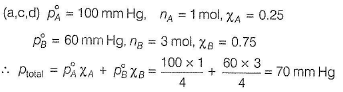But, actual vapour pressure = 75 mm Hg. Vapour pressure is increased. Force of attraction between A and B is smaller than between A and A and between B and B. Thus, more vaporisation due to decrease in boiling point, a case of positive deviation.

Test: Colligative Properties (Lowering Of Vapour Pressure) - Question 15

At 35°C, the vapour pressure of carbon disulphide (CS2)is 512 mm Hg and that of acetone (CH3COCH3)is 344 mm Hg. A solution of CS2 and CH3COCH3 in which mole fraction of CS2 is 0.25 has a total vapour pressure of 600 mm Hg. Which of the following statement(s) is/are correct?

Detailed Solution for Test: Colligative Properties (Lowering Of Vapour Pressure) - Question 15

(A for acetone, C for carbon disulphide)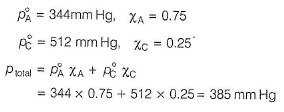But, actual ptotal = 600 mm Hg

Thus, there is positive deviation. Boiling point is lowered.
Thus, ΔH = + ve (endothermic), i.e. heat is absorbed.

Test: Colligative Properties (Lowering Of Vapour Pressure) - Question 16

Comprehension Type
This section contains 2 paragraphs , each describing theory, experiments, data, etc. four questions related to the paragraph have been given. Each question has only one correct answer out of the given 4 options (a), (b), (c) and (d)

Passage I
At 300 K, vapour pressure of pure liquid A = 575torr
and that of pure liquid B = 390torr
In binary mixture of liquids A and B with ideal behaviour, mole fraction of A in vapour phase =0.35
Q. Binary mixture contains

Detailed Solution for Test: Colligative Properties (Lowering Of Vapour Pressure) - Question 16

Let moles of A = nA and moles of B = nB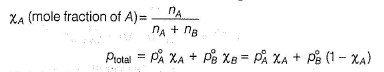Mole fraction of A in vapour state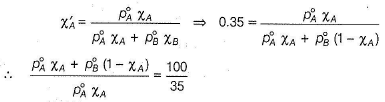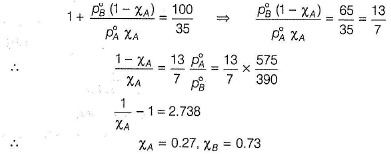Thus, A is 27 mole %.
Total vapour pressure of binary mixture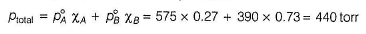Test: Colligative Properties (Lowering Of Vapour Pressure) - Question 17

Passage I

At 300 K, vapour pressure of pure liquid A = 575torr and that of pure liquid B = 390torr In binary mixture of liquids A and B with ideal behaviour, mole fraction of A in vapour phase =0.35

Q. Total vapour pressure of the binary mixture is

Detailed Solution for Test: Colligative Properties (Lowering Of Vapour Pressure) - Question 17

Let moles of A = nA and moles of B = nBMole fraction of A in vapour stateThus, A is 27 mole %.
Total vapour pressure of binary mixtureTest: Colligative Properties (Lowering Of Vapour Pressure) - Question 18

Passage II

At 40°C, the vapour pressure (in torr) of methanol-ethanol solution is represented by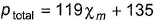where,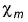is the mole fraction of methanol.

Q. Difference of vapour pressures of volatile liquids (methanol-ethanol) in pure state at 40°C is

Detailed Solution for Test: Colligative Properties (Lowering Of Vapour Pressure) - Question 18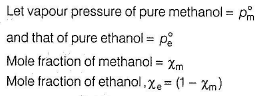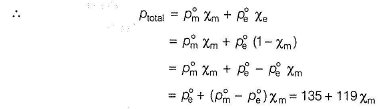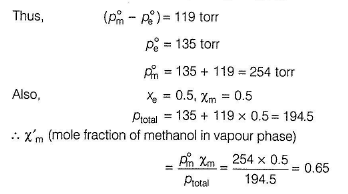Test: Colligative Properties (Lowering Of Vapour Pressure) - Question 19

Passage II

At 40°C, the vapour pressure (in torr) of methanol-ethanol solution is represented bywhere,is the mole fraction of methanol.

Q.

What is the mole fraction of methanol in vapour phase in a binary mixture with mole fraction of ethanol 0.50?

Detailed Solution for Test: Colligative Properties (Lowering Of Vapour Pressure) - Question 19

(C)Test: Colligative Properties (Lowering Of Vapour Pressure) - Question 20

Matching List Type
Choices for the correct combination of elements from Column I and Column II are given as options (a), (b), (c) and (d), out of which one is correct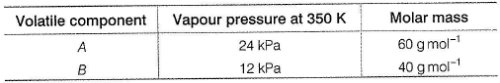100 g of binary mixture of A and B contains 60% of A. Mixture is subjected to the following operation.

Original mixture I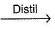II containing vapours of A and B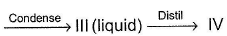containing vapours of A and B

Match the content of Column I with their values in Column II and select the answer from the codes given below.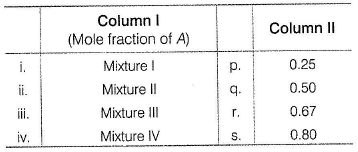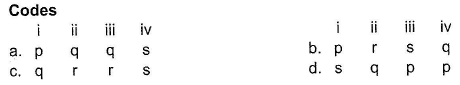Detailed Solution for Test: Colligative Properties (Lowering Of Vapour Pressure) - Question 20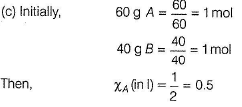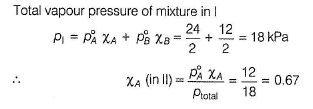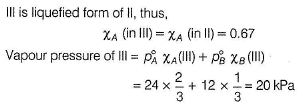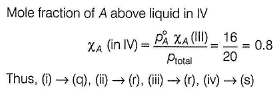## Chemistry Class 12

157 videos|385 docs|261 tests
 Use Code STAYHOME200 and get INR 200 additional OFF Use Coupon Code
Information about Test: Colligative Properties (Lowering Of Vapour Pressure) Page
In this test you can find the Exam questions for Test: Colligative Properties (Lowering Of Vapour Pressure) solved & explained in the simplest way possible. Besides giving Questions and answers for Test: Colligative Properties (Lowering Of Vapour Pressure), EduRev gives you an ample number of Online tests for practice

## Chemistry Class 12

157 videos|385 docs|261 tests

### How to Prepare for NEET

Read our guide to prepare for NEET which is created by Toppers & the best Teachers